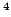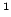Statistica Sinica 18(2008), 405-424

ON CONSTRAINED M-ESTIMATION AND ITS RECURSIVE

REGRESSION MODELS

Zhidong Bai, Xiru Chenand Yuehua WuNortheast Normal University,National University of Singapore,Graduate University of the Chinese Academy of Sciences andYork University

Abstract: In this paper, the constrained M-estimation of the regression coefficients and scatter parameters in a multivariate linear regression model is considered. Robustness and asymptotic behavior are investigated. Since constrained M-estimation is not easy to compute, an up-dating recursion procedure is proposed to simplify the computation of the estimators when a new observation is obtained. We show that, under mild conditions, the recursion estimates are strongly consistent. A Monte Carlo simulation study of the recursion estimates is also provided.

Key words and phrases: Asymptotic normality, breakdown point, consistency, constrained M-estimation, influence function, linear model, M-estimation, recursion estimation, robust estimation.